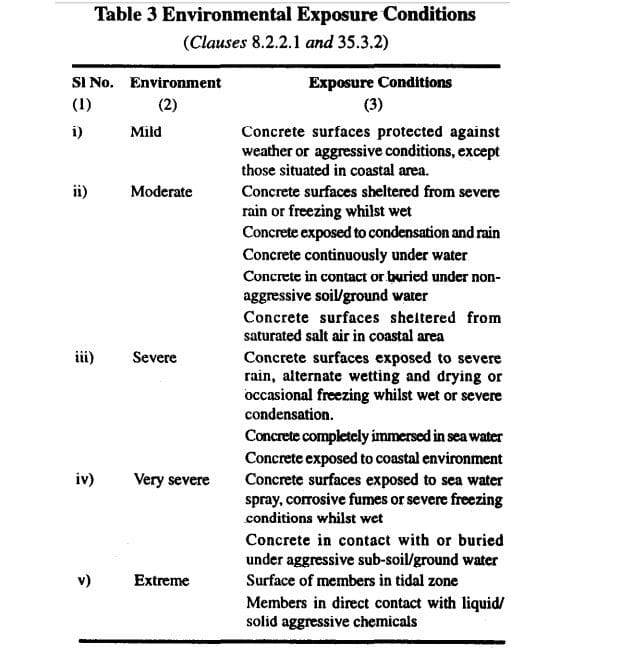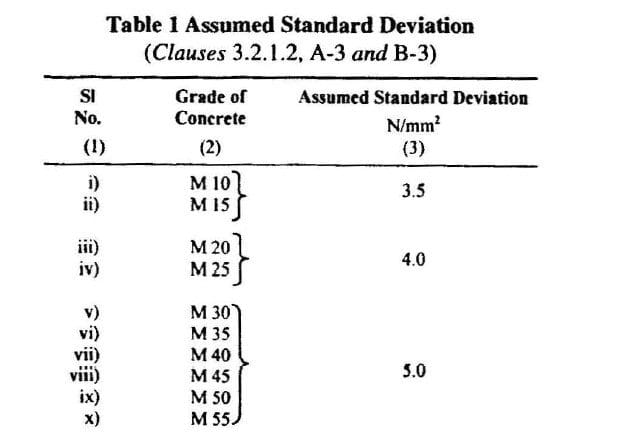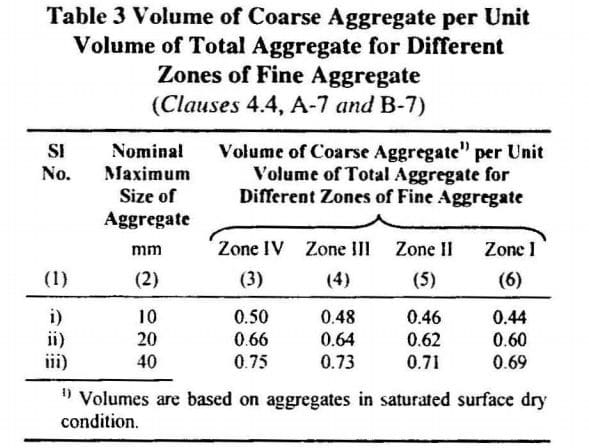# High Strength Concrete Mix Design (M60 and Higher)

Concrete mix design is a procedure of selecting the suitable ingredients of concrete and their relative proportions with an objective to prepare concrete of certain minimum strength, desired workability and durability as economically (value engineered) as possible.

Designing high strength concrete – HSC (Grades M60 and above) become a challenges in terms of selecting right proportions of conventional concrete ingredients (cement,sand and aggregate) and necessary inclusions of Chemical admixture (one or more) and Mineral admixture (one or more).

High strength concrete(HSC) has been feasible with latest generation High Range water Reducing admixture (HRWRA) and high reactive mineral admixtures like Silica Fumes, Matakaolin etc. High strength concrete becomes high performance concrete with right concrete mix design.

Applications of High Strength concrete are high rise building (typically above 30 story), high strength concrete made such projects feasible due to enhance load carrying capacity, it also allowed for the reduction of column and beam sizes. Lower dead loads result in reducing the loads connected with foundation design. Also, end user if benefitted economically since the amount of rentable floor space, increases as the space occupied by the columns decreases. It is estimated that for a 50-story structure with 1.2m diameter columns using M30 concrete can reduce column diameters by approximately 33% by using M60 concrete.

Production of HSC has to be cautiously monitored at site level to ensure the required end results.

## Mix Design of M60 Grade Concrete

We are going to illustrate a M60 concrete incorporating silica fumes,flyash,a HRWRA with other conventional conventional ingredients.

• Role Of Silica fumes: Pore Refinement (high performance) High early age and ultimate compressive strength etc.
• Role of Fly Ash: Pore Refinement (high performance), long term strength etc.
• Role Of HRWRA: water content limiting w/cm to 0.32 , *cm = cementitious content

Note here we will be using the term total cementitiuos content (Cement + flyash + Siloica fume) instead of cement content

## Primary Data for Mix Design

As we decide to go for a high strength concrete mix design, collect the following data before hand as few design stipulation are freezed on the basis of these data.

Purely governed on the local conditions, were the concrete need to be applied

a. Exposure Conditions of the structure: The general environment to which the concrete will be exposed during its service life, is categorized into five class to severity, as per IS 456.The exposure condition limit the minimum cement content content (with flyash & Silica fumes it is total cementitious content – “cement + flyash”), maximum water – cement ratio  (with flyash & flyash it is total water – cementitious ratio) and minimum grade of concrete.

As per exposure condition, you have the above data for working the first trial and arriving its mix proportions.

b. Minimum thickness of member: Size of aggregate should not be more than one-fourth of the minimum thickness of member, mostly 20 mm nominal size aggregate is suitable for most works. It is always suggested to go the maximum nominal size of aggregate to save on quantity of cement per unit of concrete.

d. Workability: Placing condition of concrete governs its workability, low – slump of 25-75 mm (lightly reinforced sections in slab, beam, column) to high –  slump of 100-150 mm (slip form, pumped concrete) .

## Illustrated example of M60 Grade Fly Concrete, concrete exposed to Severe Conditions

### (i) Stipulation for Proportioning Concrete Ingredients

(a) Characteristic compressive strength required in the field at 28 days grade designation — M 30

(b) Type of Cement : OPC 53 Grade confirming to IS 12269

(c) Type of Fly Ash : Class F

(d) Silica fumes : 20000 m2/kg (Surface area)

(e) Maximum Nominal size of aggregate — 20 mm

(f) Shape of CA — Angular

(g) Workabiltiy required at site — 125 mm (slump)

(h) Type of exposure the structure will be subjected to (as defined in IS: 456) — Severe

(i) Method of concrete placing: pump able concrete

### (ii) Test data of material

The following materials are to be tested in the laboratory and results are to be ascertained for the design mix

(a) Cement Used : OPC 53 Grade Confirming to IS 12269

(b) Fly Ash : Class F

(c) Specific Gravity of Cement : 3.15

(d) Specific gravity of Fly Ash: 2.2

(e) Specific gravity of Silica Fume : 2.2

(f) Chemical admixture(HRWRA) : Super plasticizer confirming to IS 9103

(e) Specific gravity of HRWRA : 1.1 ,solid content = 42 %

(g) Specific gravity of Aggregates

Specific gravity of Fine Aggregate (sand) : 2.70

Specific gravity of Coarse Aggregate : 2.80

(g) Water Absorption

Coarse Aggregate : 0.4%

Fine Aggregate : 1.0%

(h) Free (surface) moisture

Coarse Aggregate : Nil

Fine Aggregate : Nil

Aggregate are assumed to be in saturated surface dry condition usually while preparing design mix.

(g) Sieve Analysis

Fine aggregates : Confirming to Zone I of Table 4 IS – 38

## Step-By-Step Procedure for High Strength concrete Mix Design of M60 grade concrete

### Step-1 : Determining The target Strength for Mix Proportioning

F’ck = fck + 1.65 x S

Where,

F’ck = Target average compressive strength at 28 days

Fck = Characteristic compressive strength at 28 days

S = Assumed standard deviation in N/mm2 = 5 (Considered same as M55 grade for High Strength Concrete)

= 60 + 1.65 x 5.0 = 68.25 N/mm2

Note : Under control conditions if Target average compressive strength is achieved then at field ,the probability of getting compressive strength of 60 MPa is very high### Step-2 : Selection of Cementitious Content

As per IS 456 Table 2, the note 2 highlights that “for concrete of compressive strength greater than M55, design parameters given in the standard may not be applicable and the values may be obtained from specialized literatures and experimental results”.

We will consider the following contents to initiate first trial referring specialized literatures and our experiences

Cement = 400 kg/m3

Fly Ash = 80 kg/m3 (20% by weight of cement)

Silica Fume = 40 kg/m3 (10% by weight of cement)

Total Cementitious content = 520 kg/m3

### Step-3 : Selection of Water-Cementitious ratioi

Please note a High strength concrete need to have very low w/cm, to the tune of <0.35

Here we will restrict water – cementitious ratio to 0.32 (w/cm)

Water Content = (w/cm ) * cm = 0.32 * 520 = 166.4 say 166 kg.

### Step-4 : Finalizing Dosage of HRWA

To make a concrete workable with such a low w/cm ratio ,we need to have a HRWRA ,we will initially fix a dosage of 1% by weight of total cementitious content.

HRWRA Content = 0.01*520 = 5.2 kg/m3

### Step-5 : Correction for Water Content for Water Present in HRWA

Water content plays a very critical role in HSC, so water present in any concrete ingredient need to be corrected to maintain chosen w/cm ratio

We have 42 % solid content in chosen HRWRA, water present is 56 %

Water contributed by HRWRA is 0.56*5.2 = 2.912 say 2.9 kg.

Water Content arrived at Step 3 to be reduced by 2.9 kg.

Corrected water content = 166 – 2.9 = 163.1 say 163 kg.

### Step-6 : Proportion of Volume of Coarse Aggregate and Fine Aggregate required

From Table 3 of IS 10262- 2009, Volume of coarse aggregate corresponding to 20 mm size and fine aggregate (Zone I) = 0.60

Note 2: Incase the coarse aggregate is not angular one, then  volume of coarse aggregate may be required to be increased suitably based on experience.### Step-7 : Estimation of Concrete Mix Calculation

The mix calculations per unit volume of concrete shall be as follows:

a) Volume of concrete = 1 m3

b) Volume of cement = (Mass of cement / Specific gravity of cement) x (1/1000) = (400/3.15) x (1/1000) = 0.127 m3

c) Volume of flyash = (Mass of flyash / Specific gravity of flyash) x (1/1000) = (80/2.2) x (1/1000) = 0.036 m3

d) Volume of silica fume = (Mass of Silica fume / Specific gravity of Silica fume) x (1/1000) = (40/2.2) x (1/1000) = 0.018 m3

e) Volume of HRWRA = (Mass of HRWRA / Specific gravity of Silica fume) x (1/1000) = (5.2/1.1) x (1/1000) = 0.005 m3

f) Volume of water = (Mass of water / Specific gravity of water) x (1/1000) = (163/1) x (1/1000) = 0.163 m3

g) Total Volume of Aggregates = 1- (b+c+d+e) =1- (0.127+0.036+0.018+0.005+0.163) = 0.651 m3

h) Mass of coarse aggregates = Total volume of Aggregates X Volume of Coarse Aggregate X Specific Gravity of Coarse Aggregate X 1000 = 0.651 X 0.60 X 2.80 X 1000 = 1094 kgs/m3

h) Mass of fine aggregates = Total volume of Aggregates X Volume of Fine Aggregate X Specific Gravity of Coarse Aggregate X 1000 = 0.651 X 0.40 X 2.70 X 1000 = 703 kgs/m3

### Step-8 : Final Concrete Mix Proportions for M60

Cement = 400 kg/m3

Fly Ash = 80 kg/m3

Slica fume = 40 kg/m3

HRWRA = 5.2 kg/m3

Water = 161 kg/m3

Fine aggregates = 703 kg/m3

Coarse aggregate = 1094 kg/m3

Water-cementitious ratio = 0.32

Note-1: Aggregates should be used in saturated surface dry condition. If otherwise, when computing the requirement of water, allowance shall be made for the free (surface) moisture contributed by coarse aggregate and fine aggregate. On the other hand, if the aggregates are already dry, the amount of water mixed should be increased by an amount equal to the moisture likely to be absorbed by the aggregates. Necessary adjustments are also required to be made in the mass of the aggregates. The surface water and percentage water absorption shall be determined according to IS 2386.

Correction in water content due to absorption or moist aggregates should incorporated.

The slump shall be measured and the water content and shall be adjusted for achieving the required slump based on trial, if required. The mix proportions shall be reworked for the actual water content and checked for durability requirements.

Two more trials having variation of +/- 10% of water cementitious ratio  shall be carried out and a graph between three water-cementitious ratios and their corresponding strength shall be plotted to work out the mix proportions for the given target strength for field trials. However, durability requirement shall be met.

Two more trials having variation in fly ash % of 15 & 25 and Silca Fumes % of 7.5 and 12.5% shall be carried out to optimise flyash and silica fume content